AERODYNAMICS

The challenge in explaining aerodynamics for glider pilots is to provide the information needed to fly safely and efficiently without overloading the student with complex theories. Meeting this challenge involves using analogies and simple explanations that convey the essential information. Some of the traditional ways of doing this have been questioned recently as perhaps going so far that they are actually untrue. (See http://www.informatik.uni-frankfurt.de/~plass/MIS/mis6.html and http:// www.avweb.com/articles/liftsuck/index.html). We will try to avoid that kind of error here, but also include traditional references when they relate directly to the FAA knowledge tests.

VECTORS

It is impossible to understand or explain aerodynamics for pilots without using vectors. The term frightens some pilots, but it need not. What follows is an explanation of vectors for shoe salesmen; engineers may skip ahead if they like.

A vector is just an arrow, drawn to represent something that has magnitude and direction. All our aerodynamic vectors will represent forces. The magnitude of a force in the U.S. and a few other backward countries is measured in pounds. Usually, we are interested only in the relative magnitude of forces, so the unit of measure is not important in most cases. The length of the arrow represents the magnitude of the force. The direction that the force acts is shown by the direction that the arrow points.

Vectors are useful when we want to know the effect of combining two forces. When the two forces act in the same direction, we really don't need vectors to know their combined effect. If you weigh 170 pounds and your dog weighs 20 pounds, even a shoe salesman knows that if you get on the scale holding your dog, it will read 190 pounds. The force vectors for your weight and for your dog's weight both act downward on the scale, and we simply add them. We do need vectors when we want to know the combined effect of two forces that act in different directions.In our illustrations, the red vector represents the combined effect of the two blue vectors. We add the blue vectors by drawing a parallelogram (in most of our aerodynamic examples it is also a rectangle) with the two blue forces represented by the two blue sides. The red vector is the diagonal of the parallelogram, and its magnitude and direction represents the combined effect of the two blue vectors. The mathematical term for the red vector is that it is the "resultant" of the blue forces.

In many cases it is useful to draw the two blue vectors originating at the same point, as shown in the examples on the left. Two aerodynamic forces acting through the same point is a good example of this type of display. In other cases, where the subject may be distances or speeds, it may be better to show the vectors as sequential lines, as illustrated on the right. In that case the blue vectors are like the sidewalk you should be walking on, and the red vector is taking the shortcut. Both get you to the same place. Since opposite sides of a parallelogram are parallel and equal in length, the resulting diagonal is the same whether we choose a common starting point or a sequential start for the blue vectors. The black lines are there just to complete the parallelogram, but they add no information to the illustration, so they can be omitted if that improves the picture by removing clutter.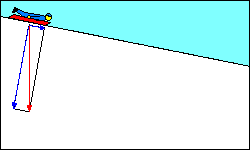Sometimes, we want to know the "components" of a single force along two different axes, or directions. The force causing your sled to move down the hill is the component of its weight acting parallel to the slope. The red vector represents the weight of the loaded sled (gravity force) acting straight down. The blue vectors represent its force components parallel and perpendicular to the slope. Now you should be able to answer the test question about what force provides the forward movement of a glider.

AIRFOILS

The Soaring Flight Manual Glossary defines an airfoil as "Any part of an aircraft (primarily the wing and tail surfaces) that deflects the air through which it moves to produce a desired reaction"(7). This definition correctly reflects the fact that in practical operation the airfoil moves through the air. In some discussions of airfoils it is more convenient to describe their interaction with the air as if the airfoil were stationary with the air moving past it, as in a wind tunnel. In either case the term "relative wind" is used to describe the relative motion of the air opposite to the direction of motion of the airfoil.

Glider airfoil cross sections vary from the complex curved surfaces of high performance wings to the "barn door" cross-section of an SGS 2-33 horizontal tail surface. All of them "produce a desired reaction by deflecting air", although some shapes are much better than others at doing so.

The term "reaction" as used here refers to Newton's third law of motion: "Whenever one body exerts a force on a second body, the second body reacts on the first with a force opposite in direction but equal in magnitude." With respect to an airfoil that says if the airfoil pushes air downward, the air pushes the airfoil upward.

While it is easy to see that the lower surface of a wing deflects air downward, it is not so obvious that the air flowing over the top of a wing also curves downward behind the wing. In fact, the stream of deflected air extends some distance above the wing as well as below.Sighard Hoerner, author of the "bible" on drag, has this to say about lift: "The lift produced by a finite-span airfoil, such as a plate for example, or an airplane wing, can physically be understood as the upward reaction to a downward deflection of a tube or cylinder of fluid having a diameter equal to the span of the lifting element." (16) Of course the boundaries of the actual "cylinder" of air are not as sharply defined as Hoerner's illustration shows them to be, but the concept may be helpful in understanding how a glider airfoil performs its function.

It is also true that the relative wind is deflected upward ahead of the wing, much like the bow wave ahead of a ship, but that can be ignored for our purposes, since the net deflection results in downwash behind the wing.

FORCES ACTING ON AN AIRFOIL

The air flowing past an airfoil exerts forces on it that are sometimes described in terms of Bernoulli's Theorem or Bernoulli's Principle: "Bernoulli's Principle states in part that 'the internal pressure of a fluid decreases at points where the speed of the fluid increases.' In other words, high speed flow is associated with low pressure, and low speed flow with high pressure." (8) Since the air flowing above a wing is moving faster than that flowing beneath it, its pressure is less and an upward force is exerted on the wing. In his classic "Stick and Rudder" published more than sixty years ago, Wolfgang Langewiesche says, "Bernoulli's Theorem doesn't help you the least bit in flying. While it is no doubt true, it usually merely serves to obscure to the pilot certain simpler, much more important, much more helpful facts." (17)

 Perhaps a simple analogy can be used to reconcile Bernoulli and the simpler "reaction to deflected air". When a marching band changes direction of travel, those on the inside of the turn take shorter steps and those on the outside take longer steps. Similarly, when an airfoil deflects the relative wind, air on the outside of the "turn" speeds up and that on the inside slows down, which according to Bernoulli's Theorem will be accompanied by lower pressure in the faster air and higher pressure in the slower air.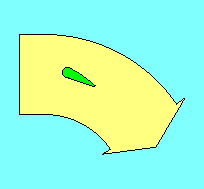LIFT AND DRAGBy now the reader probably has detected the author's slight bias against Bernoulli's Theorem as an appropriate explanation for how an airfoil develops lift. However, it still appears as the correct answer for several knowledge test questions. Therefore, if the question is concerned with what is happening above the wing, the correct answer will be Bernoulli. If the question relates to action below the wing the answer will be something about deflection of air (Newton).

The pressure distribution on the airfoil is complex and of concern to aircraft designers. Fortunately for pilots, the aerodynamic forces on the airfoil can be combined to a single force inclined to the relative wind. We don't have a name for the total force acting on the airfoil, but we do have names for its components parallel and perpendicular to the relative wind. They are "drag" and "lift", respectively.

Glider pilots should recognize two different meanings for the term "lift", depending on the context in which it is used. Aerodynamic lift, as used here, is the force on an airfoil perpendicular to its flight path, but glider pilots also refer to rising air as lift.

PARASITE DRAG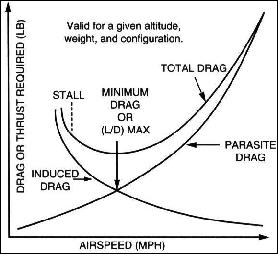We should note that drag comes in two varieties. Parasite drag is the resistance offered by the air to anything moving through it. Parasite drag of a modern sailplane wing is very low, but when the drag of the rest of the aircraft is added to it, the total is significant, particularly at high speeds since parasite drag increases with the square of speed. (i.e. double the speed and parasite drag increases four times.) Parasite drag can be further classified into form drag, skin friction, and interference drag. Glider form drag is reduced by making the frontal area of all parts as small as practical. Skin friction is reduced by reducing the surface area. Interference drag occurs where two parts join, such as the wing and fuselage, and is reduced with fairings to streamline the intersection.

INDUCED DRAG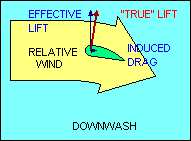The other kind of drag is induced drag and is a by-product of the generation of lift. Even if parasite drag were reduced to zero, the aerodynamic force on a wing deflecting the relative wind would not be perpendicular to the direction of flight, and the greater the wind deflection the greater the deviation of that force from perpendicular. Sometimes it is helpful to describe the total aerodynamic force (excluding parasite drag) as "true lift" perpendicular to the "average" relative wind. This explanation recognizes that the relative wind is deflected by the airfoil and its direction after deflection, called "downwash", is no longer parallel to the flight path. Thus its "average" direction lies somewhere between the flight path and the downwash angle.

In this explanation, the component of "true lift" perpendicular to the flight path is called "effective lift" and the component parallel to the flight path is induced drag. This explanation introduces new definitions of "lift" and "relative wind" which may be confusing to some students. In all other uses of these terms in this document, "lift" is defined to be perpendicular to the flight path and "relative wind" is parallel to it. In any case, it follows then that induced drag, the aerodynamic force component parallel to the flight path, increases as the amount of deflection of the relative wind increases.

CHORD, CAMBER AND ANGLE-OF-ATTACK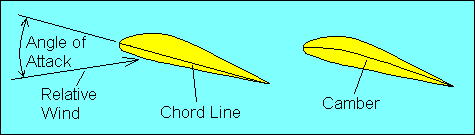There are two ways to increase the degree of deflection of the relative wind, and they require defining three more terms. A straight line drawn from the airfoil leading edge to its trailing edge is the "chord line". A curved line drawn between the same two points and midway between the upper and lower surface of the airfoil defines its "mean camber", often simply called "camber". If the term camber includes the modifiers "upper" or "lower" it is referring to the curvature of the upper or lower surface of the wing. The angle between the chord line and the relative wind is called the "angle of attack". Increasing the angle of attack or increasing the camber increases the degree to which air is deflected by the airfoil, and thus increases both lift and induced drag for a constant airspeed. Angle of attack should not be confused with "angle of incidence", which refers to the angle formed by the chord line and the longitudinal axis of the aircraft. Angle of incidence is fixed; the pilot controls angle of attack.GROUND EFFECT The fact that induced drag is directly related to the deflection of the relative wind is the reason for an improvement in glider performance near the ground called "ground effect". As a glider descends below an altitude approximately equal to one wingspan, the interaction of the deflected air and the surface results in a reduction in the angle to which the relative wind is deflected with a corresponding reduction in induced drag.

ANGLE-OF-ATTACK VS SPEEDIn wings level, unaccelerated flight the total lift force is essentially equal to the weight of the aircraft. We will defer until later a more complete discussion of forces acting on the glider in flight. For now we need only recognize that the lift force depends on the angle through which the relative wind is being deflected (downwash) and the rate at which air is moving past the airfoil. The same lift force can be created by deflecting a lot of air through a small angle or a little air through a large angle. If we assume camber to be fixed, the angle of deflection of the relative wind depends on the angle of attack, and the amount of air moving past the airfoil depends on the airspeed. Thus to keep lift constant we must associate high speed with low angle of attack and low speed with high angle of attack.

Remembering that parasite drag increases directly with the square of speed while induced drag varies inversely with the square of speed, we can see that total drag is high both at very low speeds and at very high speeds and reaches a minimum somewhere in between. The speed where this occurs is where parasite drag and induced drag are equal, and it is the most efficient speed for the aircraft in an aerodynamic sense, although it may not be optimum for any specific flight objective.

TRAILING VORTICIESWith all those aircraft up there deflecting air downward you'd think there wouldn't be any left except at the surface. That isn't true, of course, because the deflected air returns more or less to its original position after passage of the airfoil. In fact, even as the air is being deflected downward by a wing, it is escaping around the wing tips in an attempt to return to its original position, creating "trailing vortices", which resemble little tornadoes coming backward from the wing tips. They are the main constituents of "wake turbulence", sometimes incorrectly called "prop wash". It occurs behind every airfoil that is generating lift and can represent a significant safety hazard when caused by a large airplane. Since it is a direct result of the air being deflected, it is greatest when deflection is greatest, i.e. when the aircraft is heavy and slow. Glider pilots utilizing aero tow find that flight in the wake of the towplane is possible but not desirable.

STALL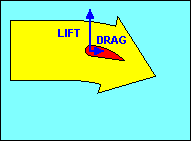Before we leave the discussion of airfoils, we should examine what happens if we continue to increase angle of attack. As you might expect there is some point where the relative wind will no longer follow the sharply curved path that the airfoil is trying to force it to follow. It is not unlike a racecar that hurtles off the track while trying to negotiate a too sharp curve. The relative wind breaks away from the airfoil, accompanied by a relatively abrupt increase in drag and a similar decrease in lift. This action is called a "stall" and is dependent only on the angle of attack. Unlike the racecar, speed has nothing to do with it, but since gliders usually have airspeed indicators but not angle of attack indicators, we recognize "stall speed" as that speed at which a glider gradually slowing down with wings level reaches the critical (stalling) angle of attack. It is important to remember that stalls can occur at any airspeed and in any attitude, even though they frequently are practiced with wings level and nose high.

The FAA isn't likely to ask about it, but a curious student may want to know why graphs depicting coefficient of lift versus angle of attack typically show a much gentler stall than the one experienced in the glider. The short answer is that the graphs are made from data collected from a model in a wind tunnel while the student's view is from a glider in the air. In the wind tunnel it is possible to hold constant all the factors other than the ones you are trying to evaluate. As a result, the graph shows coefficient of lift increasing linearly with angle of attack until it nears the stall. There it changes to a gentle curve that turns back down, with coefficient of lift decreasing as angle of attack increases, usually at a faster rate than it increased on the front side of the curve, but not as quickly as it seems in the glider.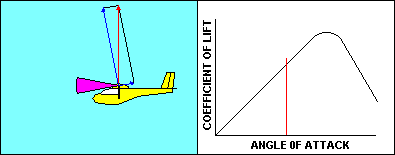As the glider approaches a stall the angle of attack and the corresponding coefficient of lift both increase pretty much the way you would expect them to, based on the graph. Speed is decreasing so that the aerodynamic resultant of lift and drag remains equal to the glider weight. Once the critical angle of attack is reached, the coefficient of lift starts to diminish and there is no offsetting speed increase, so lift decreases. The glider starts to fall, and that causes the direction of the relative wind to change. That causes an increase in angle of attack that further reduces the coefficient of lift. That makes the glider fall faster, increasing angle of attack, etc, etc. The result is that the glider in actual flight moves very rapidly down the already steeper back side of the curve. The gentle stall of the wind tunnel becomes an abrupt change in glider performance that can be fatal at low altitude.

FORCES ON THE GLIDER IN STRAIGHT FLIGHT

We have discussed forces acting on an airfoil. Now let's look at the forces acting on the whole glider.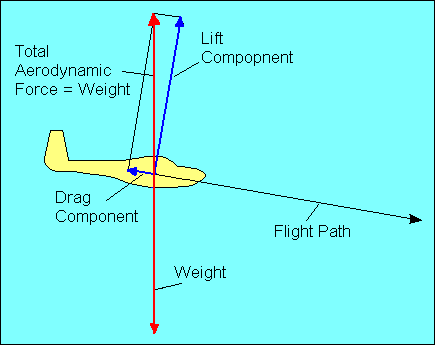In straight flight at constant speed the forces on the glider are balanced. Since gravity, the weight of the glider, acts straight down, it must be offset by an equal aerodynamic force straight up. This total aerodynamic force is the sum of all the aerodynamic forces acting on the glider and has no name. Instead, as we did with airfoils, we resolve this force into its components parallel and perpendicular to the flight path and call them drag and lift, respectively. For airplane pilots used to seeing drag offset by thrust, we could show that weight has a force component along the glide path equal to drag and a perpendicular component equal to lift. In either case the forces are balanced, but the former explanation will be used in the following discussion.

GLIDE RATIO

The angle at which the glider descends is usually called its "glide ratio", numerically equal to the horizontal distance it travels divided by the vertical distance it travels in the same time. In the following discussion we mean "glide ratio" relative to the air mass through which the glider is flying. "Glide Ratio" with respect to the ground (generally of much greater interest to the pilot in actual flight) is affected by both horizontal and vertical movement of the air mass. The explanation here is valid with respect to the ground only in "still air" conditions.If a glider travels 20 feet forward for each foot it descends, it has a 20 to 1 glide ratio. This relationship also applies to speed since there is no significant difference in horizontal speed and the speed along the actual glide path. Thus a glider flying at 40 knots airspeed and descending at 2 knots vertical speed also has a 20 to 1 glide ratio.

Once again we use the graphic vector analysis technique and show that the glide ratio is numerically equal to the ratio of lift to drag. Either of the brown angles, when added to the green angle, equals 90 degrees. Therefore, the brown angles are equal. The lift/drag ratio is a measure of the upper brown angle and the glide ratio is a measure of lower brown angle. Thus the lift/drag ratio is numerically equal to the glide ratio. (When glider pilots say "L over D" they are referring to this ratio and are not trying to yodel.) This means that a 1,000-pound glider flying at a 20 to 1 glide ratio is experiencing a total drag of 50 pounds. We have assumed here as we did with speed that the difference between lift and weight is negligible so long as the angles are small. Even at a 10 to 1 glide ratio the lift required to support a 1,000-pound glider is 995 pounds, a difference of only one half of one percent. The remaining 5 pounds is supplied by the vertical component of drag.

GLIDER AXES OF MOTIONLONGITUDINAL LATERAL VERTICAL AXIS AXIS AXIS ROLL PITCH YAW

Our experience moving about on the ground only required turning right and left. In the air we have two additional degrees of freedom. We define these in terms of three axes and the motion of the aircraft about them. The "longitudinal" axis runs from the nose to the tail of the aircraft and motion about it is called "roll". The "lateral" axis runs from wing tip to wing tip and motion about it is called "pitch". The "vertical" axis is perpendicular to both the others (note that it is really "vertical" only in level flight) and motion about it is called "yaw". All three axes intersect at the center of gravity (CG) of the glider.

STABILITY

Stability, the tendency to return to the original position after being disturbed, must be provided for all three axes of the aircraft. When we use the term "stability" here we mean "positive static and dynamic stability". That sounds more ominous than it really is. "Static stability means that if the airplane's equilibrium is disturbed, forces will be activated which will initially tend to return the airplane to its original position." (8)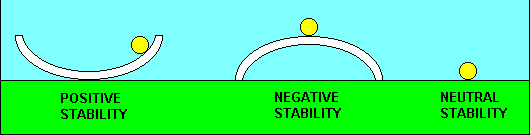Stability can be illustrated by the old "lug nut and hubcap rule", which originated back in the days before wheel covers and when changing a tire was part of learning to drive. If the lug nuts are placed in the hubcap with its open side up, the lug nuts tend to return to the center when they are disturbed. That is positive stability. If you place the lug nuts on the rounded top of the hubcap, they will roll off with the slightest provocation. That is negative stability. If you already lost the hubcap and must place the lug nuts on the ground, they tend to stay pretty much anywhere you put them. They neither tend to return to a more stable place nor move farther away from it. That is neutral stability.In our example of positive static stability the lug nut overshot the center of the hubcap before it started back the other way. Each time it did this, however, the amount of overshoot was less until it finally stopped in the center. That is an example of positive "dynamic" stability. If the overshoot had grown larger each cycle until the lug nut escaped the hubcap and disappeared, that would have been an example of positive static stability with negative dynamic stability. Lug nuts don't do that but aircraft can. An example might be "flutter", where a component of the aircraft fails due to rapid uncontrolled oscillation.

FLUTTER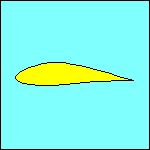In our illustration an airfoil with an attached control surface, such as an aileron, is displaced upward by a gust. Because the center of gravity of the control surface is aft of its hinge point, the surface lags behind the airfoil as it rises. This downward deflection of the control surface increases the upward aerodynamic force on the airfoil and forces it even higher. If this example represented a wing, the wing would bend upward until its resistance to bending equaled the upward force. Then the wing would start back down and the aileron would deflect upward, again due to its cg location aft of the hinge point. Now the aerodynamic effect of the deflected aileron is adding to the downward force. The whole process reverses again when the airfoil reaches its lowest position, and it continues to flutter up and down until it departs from the aircraft. The chances of this happening can be greatly reduced if not eliminated by placing counterweights on the other side of the control surface hinge line to move its center of gravity near the hinge.

Flutter is usually associated with high true airspeed. "Flutter below red-line speed can be caused by looseness in control cables, connections, hinges, or play in the wing or empennage attachments, or even by the pilot's action." (12)

Although there is interaction between stability about different axes, we will describe the primary contributor to stability for each axis as if they were independent.

DIRECTIONAL STABILITY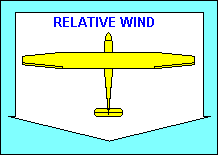"Directional" stability is provided about the vertical axis by the vertical tail surfaces. They act essentially the same way feathers on an arrow do, to make the aircraft point in the direction it is moving (i.e. into the relative wind). The tail surfaces are airfoils and produce a "lift" force when they are inclined to the relative wind, just as the wing does. In this case the force is horizontal and pushes the glider tail back into alignment with the relative wind.

LATERAL STABILITY

"Three factors that affect lateral stability are: Dihedral; Sweepback; Keel Effect." (8) All depend on the fact that an aircraft whose wings are not level will tend to move in the direction of the low wing because lift is tipped in that direction.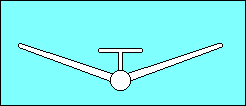Dihedral, raising the wing tips above their roots, provides lateral stability about the longitudinal axis. Aircraft movement toward the low wing combined with dihedral increases the angle of attack of the low wing and decreases the angle of attack of the high wing. This unequal lift on the wings returns the aircraft to a wings level attitude. The location of the wing on the fuselage contributes to its effective dihedral. A low wing aircraft may need as much as 5o more dihedral than a high wing aircraft for the same effect.The upwind (low) wing of an aircraft with sweepback produces more lift than the downwind (high) wing because lift depends on the airspeed perpendicular to the wing's span-wise axis. The relative wind strikes the low wing more directly than the high wing and the lift differential tends to return the aircraft to wings level flight. Some gliders utilize forward sweep of their wings to get the desired relationship between the center of gravity and the center of lift. When they do so it is necessary to increase dihedral to compensate for the negative effect of forward sweep on lateral stability.

"Keel" effect might better be called "rudder" effect. It refers to the rolling moment caused by the effect of the relative wind on the side of the fuselage. A significant part of the rudder area is above the center of gravity of the aircraft, and the relative wind striking it tends to roll the aircraft back to wings level flight.

LONGITUDINAL STABILITY

Directional and lateral stability are important but they are determined by the designer of the aircraft and beyond the control of the pilot. Longitudinal stability, about the lateral axis, is controlled by the pilot, so it is even more important to understand what contributes to it and how to manage it. This involves understanding moments, another subject that may seem more complex than it really is. A moment is a twisting action, and if you ever tried to loosen a nut you applied a moment to it. A moment is measured by the product of a force and a distance (called an arm in our examples). We will use pounds for force and inches for distance, so a moment will be measured in pound-inches, representing the product of multiplying the force times the arm. The moment you applied to the nut could be increased by pushing harder on the wrench (increase the force) or getting a longer wrench (increase the arm).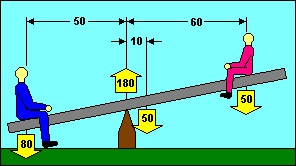Longitudinal stability is often compared to a seesaw. Several of the knowledge test questions use an illustration that looks a lot like a seesaw except that boxes are substituted for boys. In our example, the seesaw is balanced when the moments on each end are equal. We can do that with a big boy on the short end and a small boy on the long end. The 80 pound boy times his 50 inch arm balances the sum of the 50 pound seesaw times its 10 inch arm plus the 50 pound boy when he moves out to 70 inches. (80 x 50) = (50 x 10) + (50 x 70). Note also that the sum of the forces acting upward is equal to the sum of the forces acting downward. (180) = (80 + 50 + 50).

A typical test question might ask how far to move the small boy to balance the seesaw. You know that the left side total moment is 80 x 50 = 4,000 lb-in. To balance the seesaw, the small boy needs to contribute 4,000 - (50 x 10 = 500 for the seesaw) = 3,500 lb-in. Since he weighs 50 pounds, he needs an "arm" of 3,500 / 50 = 70 inches. He started at 60 inches, so he needs to move 10 inches to the right.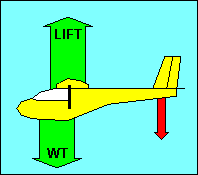If we substitute a glider for the seesaw, we can see that the moment problem is essentially the same. Instead of the large boy, we substitute the weight of the loaded glider. We can treat that weight as if it were concentrated at the glider "center of gravity" (CG). The fulcrum where the seesaw was balanced has been replaced by the "center of lift" of the glider wing. Sometimes referred to as the center of pressure, this is the point where the sum of the aerodynamic forces on the wing can be treated as a single force. It is not the same as the "aerodynamic center". The small boy is replaced by the aerodynamic force on the glider's tail surfaces, shown in red in our illustration. Common sense tells you that the force on the tail should be up like the wing. It also tells you that the earth is flat. Neither is correct. In most flight conditions, the force on the tail is down, and that is how we obtain longitudinal stability.

In unaccelerated flight, the down force on the tail is just enough to balance the glider CG. If the glider is pitched down it accelerates to a higher speed. The higher speed produces a greater down force on the tail, which returns the glider to the original pitch attitude. Similarly, if the glider is pitched up, the lower speed reduces the tail force and allows the glider to return to its original attitude. It is apparent then that the CG must be ahead of the center of lift to obtain positive longitudinal stability. In fact, stability increases as the CG moves forward and it decreases as the CG moves aft.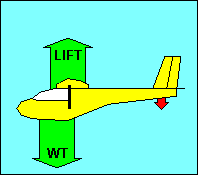Note that movement of the CG must be accompanied by a change in the down force on the tail to keep the moments balanced. Moving the CG forward increases its arm and thus increases its moment. The arm of the tail surfaces is fixed, so to balance the increased moment of the CG, the tail force must increase. That increases the apparent weight that the wing lift must support. "An airplane with forward loading is 'heavier'... than the same airplane with the center of gravity further aft. ..This requires a higher angle of attack of the wing, which results in more drag and, in turn, produces a higher stalling speed. With aft loading and 'nose-down' trim, the tail surfaces will exert less down load, relieving the wing of that much wing loading and lift required to maintain altitude. The required angle of attack is less, so the drag is less... Theoretically, a neutral load on the tail surfaces in cruising flight would produce the most efficient overall performance ...but would also result in instability." (11)
The forward and aft limits of the CG are established by the designer and must not be exceeded by the pilot when loading the aircraft. The aft limit is based on stability and exceeding it can make recovery from stalls and spins difficult or impossible. It also produces light control forces that may be difficult for the pilot to handle. The forward CG limit is usually based on landing considerations where the ability to raise the nose is essential.

WEIGHT AND BALANCE

As the preceding section indicated, the pilot has a responsibility to be sure that the glider is within weight and balance limits. Calculating "weight and balance" is easy for a glider because there are few variables. In practice it is usually sufficient to consult a chart or placard to verify that the glider is within acceptable limits, but pilots should understand the underlying calculations even if they do not need to use them very often (maybe when they take the practical test).The acceptable range for the CG is specified as minimum and maximum distances from an arbitrarily selected "datum" point, usually near the front of the glider. The weight of the empty glider and its distance from the datum (called "arm") is provided, as well as the distances ("arms") from the datum to each occupant. Moments must be calculated for the empty glider and each occupant. If the sum of these moments is divided by the total weight, the result will be the distance from the datum to the loaded glider CG.

Some of the knowledge test questions involve adding or removing weights and determining the effect on CG location. Although these can be solved by a complete recalculation, the following formula can also be used:

```       (Weight Added or Removed)          (Change in CG Location)
-------------------------  =  -----------------------------------
(New Total Weight)         (Distance Between Weight and Old CG)
```

For example: If the CG of a glider at 900 lbs is located at station 80, and you add 100 lbs at station 70, then 100/1,000 = (Change in CG)/10. The change in CG is 1, and since the weight is added ahead of the old CG, the new CG is located at station 80 - 1 = 79.

If the weight changes are permanent the aircraft weight and balance records must be revised to reflect the new empty weight and its arm and/or moment.

If the CG is within the specified limits the glider meets the "balance" requirement, but still could be outside the "weight" limit. This maximum gross weight limit is based on the structural strength of the glider. Pilots usually sooner or later observe that the wings typically don't fall off an overloaded glider. This should not be interpreted that weight and balance is just something you need to know to pass a test. Overloading a glider uses up some of the safety factor that was built into the glider to allow for stresses that can result from maneuvering or gusts. Even if you are willing to take that risk, remember that you are operating illegally and probably have invalidated your insurance.

GLIDER CONTROLS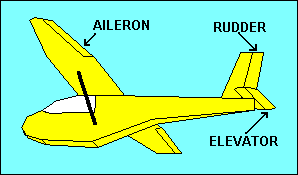Stability of a glider is good because it allows pilots to scratch their noses or get a drink from their canteens without losing control of the aircraft, but frequently they want to cause the glider to do something opposing stability, like turn. If stability were our only objective, airfoils would not need movable surfaces such as ailerons on the wings, the elevator on the horizontal tail or the rudder on the vertical tail. These provide for control of roll, pitch and yaw, respectively. They achieve control by changing the camber of the airfoil to which they are attached, which in turn changes the lift and drag of that airfoil.

A right aileron deflected downward increases lift for that wing, rolling the glider to the left. Right rudder causes air to be deflected to the right, resulting in a horizontal "lift" force on the tail to the left, yawing the nose to the right. Up elevator increases the downward "lift" force on the tail, pitching the glider nose up. Selecting the pitch attitude to which the glider will return without the application of elevator control is accomplished with pitch trim. In gliders this usually utilizes either an adjustable spring attached to the elevator control mechanism or a small adjustable tab on the elevator surface. When the trim tab is deflected downward aerodynamic forces push the elevator up, which is equivalent to applying a backward force on the control stick, either manually or with a spring type trim. The result is a pitch up and stable flight at a lower speed.

Our illustration shows a common arrangement for the horizontal tail on an aircraft, in which there is a fixed surface called the stabilizer and a movable surface called the elevator. On some aircraft these are combined in an all-moving surface called a stabilator. V-tailed aircraft combine the function of rudder and elevator in a pair of ruddervators. Horizontal tail surfaces can be mounted at the top of the vertical tail as a T-tail, or midway up as a cruciform tail, or even in front on a canard design.

FLAPS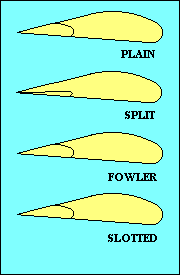While we are discussing control surfaces we should also mention the flaps. Flaps come in a variety of types, as shown in this illustration. Their purpose is to change the camber of part of the wing and sometimes also to increase wing area. Both increase lift for a given speed and usually also increase drag, but since lift has to be equal to weight, the practical effect is to allow flight at a lower speed. The added drag has the effect of reducing the glide ratio, useful in landing. Flaps generally reduce stalling speed, another benefit when landing. That feature is also good when you need to fly slowly as when following a slower aircraft in the pattern, or when you are thermalling. In addition to flaps on the wing trailing edge, some aircraft also have flaps, slats or slots at the leading edge. They increase camber and/or delay stall by providing a route for higher-pressure air below the wing to add energy to the boundary layer of air on the upper surface.

It may be of interest, since it makes a good test question, to distinguish between stability, maneuverability, and controllability. Stability is the inherent quality of an aircraft to correct for conditions that disturb its equilibrium. Maneuverability is the quality that permits it to be maneuvered easily and withstand stresses imposed by maneuvers. Controllability is the capability to respond to the pilot's control with regard to flight path and attitude.

NEWTON ON GLIDERS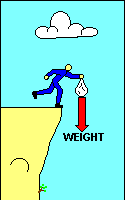Before discussing the application of these controls to maneuvers, we should review Newton's first and second laws as they apply to gliders. If the forces on a glider in flight are balanced it will continue moving in a straight line at a constant speed. We refer to this as a "steady state" condition. If the forces on the glider are unbalanced it will move in the direction of the net force and accelerate until the forces are again balanced. We refer to this condition as "transient", the change from one steady state to another. Newton's laws apply to everything. If a sick sack is dropped it will accelerate downward due to the unbalanced force of gravity until air resistance builds a drag force equal to its weight. It then assumes a steady state condition at that speed with weight and drag equal. (Similarly, its initial horizontal speed, if any, decreases due to unbalanced horizontal drag until both speed and drag are essentially zero.)

A more practical application of this principal is when a pilot applies back-pressure on the stick. That increases the angle of attack and so increases lift and induced drag. Lift, now greater than weight, accelerates the glider upward. Drag, now greater than thrust, slows the glider. When the forces again become equal, the glider will be in a shallower glide (assuming the operation is conducted at a speed greater than the best glide speed) and at a lower speed.

TURNSIf we want to change the direction of flight (i.e. turn the glider) we must apply a force in the direction we want to go. If we were driving a car we could point it in that direction and the friction between the tires and the road would provide the turning force unless we were driving on ice. Since air is even slipperier than ice, pointing the glider in the new direction won't work very well. The strongest controllable force we have in the glider is lift. In straight flight lift is pointed straight up, when viewed from the front or rear. If we roll the glider so that the lift is inclined to one side we have a horizontal component of lift to act as the necessary force to change our direction of flight. Because the vertical component of lift still needs to equal weight, the total lift, represented by the red vector in the illustration, must be increased. This usually is accomplished by increasing the angle of attack but the glider left alone will do it by sacrificing altitude for speed. "Sometimes during early training in steep turns, the nose may be allowed to get excessively low resulting in a significant loss of altitude. To recover, the pilot should first reduce the angle of bank with coordinated use of rudder and aileron, then raise the nose of the airplane ..with the elevator." (9)

The unbalanced force due to the horizontal component of lift causes the glider to accelerate toward the low wing, but turning involves yawing motion combined with this lateral movement. Here, directional stability comes in to help. The glider really doesn't "like" to move sideways and yaws to keep its fuselage more or less aligned with its direction of travel. Note that turning does not require the rudder; a fixed vertical tail will provide the yawing action described here, but as we shall soon see, there are rudder functions associated with turning efficiently.

Before leaving this description of turns we should review the relationship between speed, bank angle, and turn radius. One formula that relates them is: radius (ft) = speed (kts) squared divided by (11.26 times the tangent of the bank angle.) This indicates, for example, if you double the speed while maintaining the same bank angle, you increase the radius four times. That would also increase the circumference of the turn four times, and although the speed is doubled, it takes twice as long to complete a circle. Thus the turn rate (in degrees per second) is only half as much. To increase turn rate, bank more steeply and/or slow down. (ROT = [1091 times the tangent of the bank angle] divided by the speed) Of more interest to glider pilots circling in a thermal, the turn radius can be decreased the same way.The turn is a more complex maneuver than the preceding description suggests. While the glider is rolling into the turn (and also while it is rolling out) the increased lift on the rising wing is accompanied by an increase in induced drag which causes "adverse yaw", a yawing motion opposite from the intended turn direction when rolling in. The only function of the rudder in turn entry and roll out is to offset this adverse yaw. Our illustration shows banking for a left turn without using rudder - the nose yaws right. "If the nose starts to move before the bank starts, rudder is being applied too soon. If the bank starts before the nose starts turning, or the nose moves in the opposite direction, the rudder is being applied too late." (9) Adverse yaw is greater for gliders than for most airplanes because the long wing span provides a longer lever arm for the force to act through. It is usually much more noticeable at low speeds because the wing is already operating at a high coefficient of lift. The coefficient of induced drag varies with the square of the coefficient of lift, so the same lift increase due to aileron deflection results in greater adverse yaw at low speed than at high speed.

OVERBANKING AND YAW IN A TURN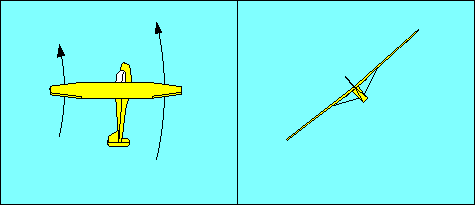Once the turn is established the wing on the outside of the turn is traveling faster than the one on the inside because it is traversing a larger circle in the same time. Since both lift and drag increase with speed, they are greater for the outside wing and result in an "overbanking tendency" and "yaw away from an established turn", respectively. Note that this yaw is not the same as adverse yaw, but it is corrected the same way, with rudder to keep the fuselage aligned with the direction of flight. Overbanking is corrected with aileron opposite the direction of turn. Both these phenomena are much more evident in gliders than in other aircraft because the glider's longer wings provide a greater speed difference between the wing tips. They are a function of wingspan and turn radius. The formula for turn radius in feet is:

r = V2 / (11.26 x Tangent of Bank Angle)
where V is speed in knots. Thus, turn radius varies with the square of speed. Rate of turn, in degrees per second, increases with bank angle and decreases with speed. Since turn radius is affected by both bank angle and speed, overbanking and yaw away from the direction of turn are most obvious at relatively steep bank angles at normal speeds and at even shallow bank angles at very low speeds.

When we discussed stability we noted that a well-designed aircraft tends to return to wings level flight when disturbed about the longitudinal axis. This is just the opposite of what happens in overbanking. When the opposing forces of stability and overbanking are equal the aircraft remains in a banked turn without aileron displacement. That is defined as a medium bank turn. In a shallow turn stability dominates and the aircraft may need aileron in the direction of turn to maintain the bank angle. In steep turns overbanking dominates and aileron opposed to the turn is required. For most gliders, shallow and medium bank turns, by these definitions, occur at very small angles.

 We have shown that it is necessary to increase total lift when turning. We can utilize the graphic vector technique to find that for a 60-degree bank, the total lift must equal twice the weight of the glider. The ratio of the total lift produced by the wing to the total glider weight is called "load factor".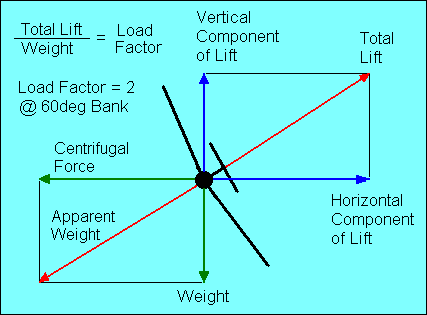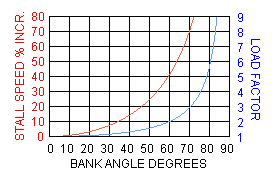As shown in this graph, at an angle of bank of slightly more than 80o, the load factor exceeds 6. The stall speed increases as the square root of the load factor; at a 60-degree bank the load factor is 2 and the stall speed increases 41%. All other performance speeds, which we will discuss later, are affected similarly. Note that load factor depends only on bank angle; it is independent of speed.

CENTRIFUGAL FORCE, CENTRIPETAL FORCE, AND LIFT

An old trick, used by young men who had just learned to drive, was to make a sharp right turn so the young lady beside them would press against them. Less romantic observers would say it was only centrifugal force causing the lady to snuggle. Perhaps a more accurate explanation is that the turning vehicle pressed against the young man, who in turn pressed against the young lady, to cause all of them to turn right. Once more we look to Newton for the answer. Indeed, turning a car or glider or two amorous young people does require a force to be applied in the direction we want to turn. The generic name for that force is "centripetal force", and according to Newton's third law, it will be accompanied by an equal but opposite reaction, which we call "centrifugal force".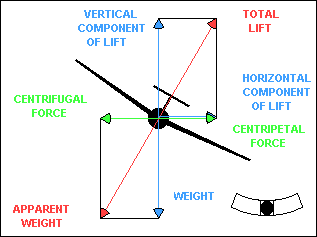In a coordinated banked turn, centrifugal force is equal to the horizontal component of lift. The resultant of centrifugal force and weight, shown in red in the illustration, is the apparent weight of the glider, which must be supported by total lift. If the turn is not coordinated, centrifugal force will not be equal to the horizontal component of lift. However, it must be equal to the centripetal force or Newton is wrong. The explanation is that the glider fuselage contributes to centripetal force when the glider is slipping or skidding in a turn. The fuselage, acting as a relatively inefficient airfoil when it is inclined to the relative wind, produces a horizontal "lift" force that can add to or subtract from the effect of the horizontal component of "wing" lift.

We defined "load factor" as the ratio of total wing lift to aircraft weight and noted, for example, that a sixty-degree bank produces a load factor of two. That is strictly true only in level flight, something a glider cannot sustain. Since lift is slightly less than weight in straight, gliding flight, the load factor in gliding turns is also slightly less than it would be in level flight at the same bank angle. However, that reduction is typically less than one percent. Most gliders do not have "g" meters to measure load factor, but the pilot should feel a significant increase in "g" force in a steep turn. If not, then either the bank is not as steep as the pilot thinks it is or the turn is not coordinated. A common error for airplane pilots, unaccustomed to the need for rudder in an established turn, is to slip in steep turns. The pitch and bank attitude look fine, but the yaw string is displaced and there is little or no evidence of "g" force.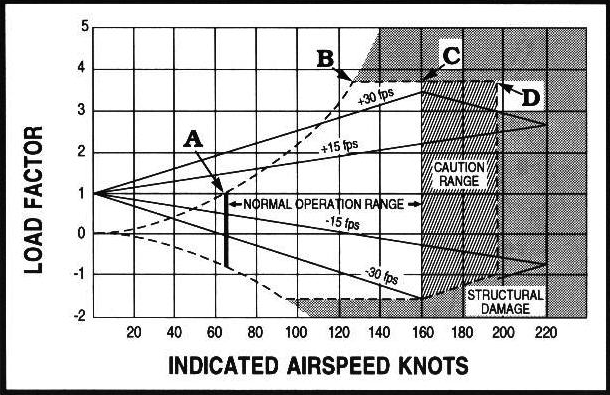This diagram shows the relationship between airspeed and both positive and negative load factors for a typical aircraft. We will limit our discussion to positive load factors since they are the ones you are most likely to encounter unless you are flying inverted. The curved dashed lines indicate the maximum load factor possible for each speed. Point "A" represents the stall speed at one "g"; what we usually think of as stall speed. Point "B" is the maneuvering speed. It occurs where the upper dashed line intersects the design limit load line for the aircraft. In this case it is about 3.8 "g". The aircraft will stall before it can reach any point above the dashed line, no matter how abruptly the controls are applied.

Abrupt control input is not the only way that the angle of attack can be suddenly increased. Vertical wind shear changes the direction of the relative wind and can produce an abrupt increase in angle of attack just as if the pilot pulled back on the stick. This possibility is covered in the chart by the sloping lines labeled 15 and 30 fps. The chance of encountering a gust greater than 30 fps is slim, so until you reach point "C" a gust is not likely to overstress the aircraft by producing a load factor greater than it was designed for. At speeds greater than point "C" you really need to be flying in smooth air where you are pretty sure you won't find a 30 fps or greater vertical gust. That's why it's called the "Caution Range". Point "D" is the "Never Exceed Speed", and it should not be approached except in smooth air.

SLIPSWhen the glider wings are level, lift and weight are essentially equal and generally constant. If lift is fixed and the glide ratio is numerically equal to the lift/drag ratio, we can change the glide ratio only by changing drag. One way to increase drag is to slip the glider. We have just discussed slips in a turn, which can be useful, but straight-line slips are probably more common and have more applications. We initiate a slip by lowering one wing, just as we do to initiate a turn. To prevent the yawing part of a turn, we apply sufficient opposite rudder to achieve a straight-line course. If you are wondering what happened to the horizontal component of lift, it is offset by an equal but opposite horizontal "lift" force, created by the fuselage, which is now at an angle to the relative wind.

Straight-line slips come in two varieties, although the glider doesn't know the difference. In a sideslip the glider maintains its original heading and slips to one side, usually to compensate for a crosswind. In a forward slip the glider maintains its original course but changes its heading, usually to steepen its glide path. "Normally, forward slips are performed with dive brakes or spoilers fully open." (7)

SPIRAL DIVE

As bank angle approaches 90 degrees the load factor could exceed structural limits of the aircraft unless the wing stalls first, due to the increase in stall speed that accompanies an increase in load factor. Since the glider's natural tendency is to increase speed by diving during a turn, an inattentive pilot could allow the aircraft to enter a "high-speed spiral dive" in which the glider's speed exceeds stall speed even at steep bank angles. The result easily could be structural failure of the glider. The spiral dive is recognized by high speed and high load factor (g force). Recovery requires leveling the wings before carefully recovering from the dive to avoid overstressing the aircraft.

SPINS

If the glider stalls in a turn it could enter a spin, which looks a lot like a spiral dive, but is significantly different. In a spin the wing is stalled and the load factor is approximately equal to one, but one wing is stalled more than the other, providing what is called "auto-rotation" about the longitudinal axis.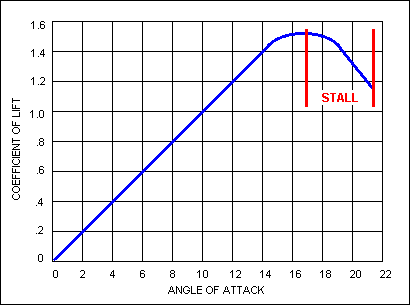Wing lift is a function of angle of attack as shown in the accompanying illustration. At normal values of angle of attack the coefficient of lift increases with angle of attack. At values greater than the critical (stalling) angle the coefficient of lift decreases as the angle of attack increases. As the spinning glider rotates about the longitudinal axis the descending wing angle of attack is increased, stalling it further. The rising wing angle of attack is reduced, making it less stalled.

"If the airplane is slipping toward the inside of the turn at the time the stall occurs, it tends to roll rapidly toward the outside of the turn as the nose pitches down because the outside wing stalls before the inside wing. If the airplane is skidding toward the outside of the turn, it will have a tendency to roll to the inside of the turn because the inside wing stalls first. If the coordination of the turn at the time of the stall is accurate, the airplane's nose will pitch away from the pilot just as it does in a straight flight stall, since both wings will stall simultaneously." (9) The glider usually spins with the nose low but if it has been loaded with the CG far aft, it may develop a "flat spin" from which recovery may not be possible.

"In the absence of the manufacturer's recommended spin recovery procedures and techniques, the following spin recovery procedures are recommended. 1-REDUCE POWER; 2-POSITION AILERONS TO NEUTRAL; 3-APPLY FULL OPPOSITE RUDDER AGAINST THE ROTATION; 4-APPLY A POSITIVE AND BRISK STRAIGHT FORWARD MOVEMENT OF THE ELEVATOR CONTROL FORWARD OF NEUTRAL TO BREAK THE STALL; 5-AFTER SPIN ROTATION STOPS, NEUTRALIZE THE RUDDER; 6-BEGIN APPLYING BACK-ELEVATOR PRESSURE TO RAISE THE NOSE." (9)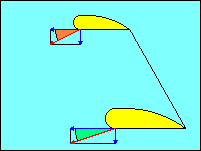In this illustration the horizontal blue vectors represent horizontal motion of the wing tips. The vertical blue vectors represent their vertical motion. The red vectors are the resultants of the pairs of blue vectors and represent the flight paths of the wing tips into the relative wind. The use of rudder to stop rotation in a spin or to pick up a low wing during a stall to prevent spinning is effective because the yawing motion increases the forward speed of the low wing. Since the vertical speed of the wing is unchanged, this reduces the wing angle of attack (shown in green). Just the opposite happens for the high wing (shown in brown). Since the wings are stalled, reducing angle of attack increases lift, the reverse of the normal, unstalled relationship, and increasing speed always increases lift. Use of ailerons to pick up a stalled wing may actually have the opposite effect since the increase in camber tries to bend the relative wind more just as increasing angle of attack does.

It should be noted that many aircraft incorporate "wash out" in their wings and other design techniques to cause the wing to stall first at the root so that aileron effectiveness is preserved. For example, although an elliptical planform (the shape of the wing when viewed from above) is the most efficient, a rectangular planform offers better stall characteristics because it tends to stall first at the wing root. However, use of rudder for roll control in stalls is a good habit to apply in any aircraft.

Spin training is not required except for flight instructors, but many instructors teach it to pilots anyway. Many trainers are difficult to spin, but spin entry and recovery can be demonstrated by applying full rudder in the desired direction of spin while holding full up elevator. Then execute the recovery as soon as the spin is recognized. "The objective of a cross-control stall demonstration maneuver is to show the effect of improper control technique and to emphasize the importance of using coordinated control pressures whenever making turns." (9)

MANEUVERING SPEED

In contrasting spins and spiral dives we observed that we could not overstress the aircraft if the wing stalled before it reached a limiting load factor. The stall speed corresponding to the maximum allowed load factor is called "maneuvering speed" and at this or lower speeds, abrupt control inputs will result in a stall before the load factor reaches excessive values. This is an appropriate speed limit in strong turbulence to avoid overstressing the aircraft. Since stall speed increases as the square root of load factor, an abrupt pull up from a dive can produce a stall as well as overstress the aircraft.

Maneuvering speed, and all other performance speeds, varies with the weight of the aircraft. If only one value is given in your aircraft manual, it probably is for maximum gross weight. Although it may seem illogical, maneuvering speed is lower for weights below maximum. You might think that it would be easier to break the wings off a heavy glider than a light one. The explanation is that other parts of the aircraft, such as the seat you are sitting on, are also designed for the maximum allowed load factor. The lighter aircraft will respond to a given gust with a greater load factor than the heavier aircraft, thus subjecting all components to a higher g force. The solution is to fly slower if you are below maximum gross weight. If your manual doesn't tell you how much slower, reduce maneuvering speed by half the percent you are below maximum gross weight (i.e. If you are 20% below max weight, reduce maneuvering speed by 10%).

EFFECT OF DRAG ON GLIDE RATIO

Recognizing that lift essentially equals weight in straight flight then the only variable affecting glide ratio is drag. To improve glide ratio we must reduce drag. Induced drag can be reduced by increasing "aspect ratio" (wing span divided by average chord). Parasite drag can be reduced by making glider components as small and streamlined as possible. If we wish to steepen our glide path, i.e. reduce the lift/drag ratio, we must increase drag. There are several ways to do that; one, as we have already seen, is to establish a slip. It is obvious that a fuselage creates more drag when it is not aligned with the relative wind.

High drag devices such as drag chutes are effective at reducing lift/drag ratio, but provide limited control. Spoilers and dive brakes can be incrementally deployed and retracted, providing excellent glide path control. Note that spoilers, so named because they spoil lift, actually steepen the glide path through their effect on drag. The lift they spoil on one part of the wing must be made up by the rest of the wing, since steady state flight requires that lift equal weight.

Similarly, high lift devices, usually limited to flaps on gliders, when used to steepen the glide path, do so because they increase drag. They do allow lift equal to weight to be obtained at a lower speed (useful when thermalling) and they usually lower stall speed, also. Positive flap deployment is associated with slow speed, but some high performance gliders also provide for negative flap deployment to improve performance at high speed.

Spoilers can also be utilized when the glider is on the ground, either parked or moving, to minimize the effect of strong winds.

GLIDER POLAR CURVE

The most frequently used way to vary glide ratio is by varying speed. Remembering that induced drag increases at low speeds and parasite drag increases at high speeds, we see that a glider flying very slowly or very fast has a relatively poor glide ratio. If we plot sink rate versus airspeed for a glider we obtain a curve called the glider "polar". The term "polar" comes from the fact that in its purest form we would be plotting speed versus glide angle. Since the angles are so small, we can get a more readable graph by converting to rectangular coordinates and expanding the vertical scale. The resulting curve looks a lot like the total drag curve turned upside down for obvious reasons.

If graphs look like way too much math for you, maybe the following explanation will help.

Suppose we have four Schweizer SGS 1-26 gliders, identical except for color. For our test, we will line them up and fly them at the speeds and sink rates in the following table:

 Glider Airspeed Sink Rate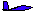26 Knots 31 Knots 42 Knots 62 Knots 1.8 Knots 1.6 Knots 1.9 Knots 3.2 Knots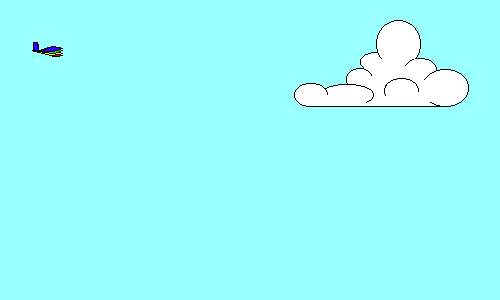If the four gliders start together and proceed in the same direction at their respective speeds and sink rates, a side view will look like this. After one minute, their positions relative to their starting points will be shown by the distance graph (for simplicity we will let one knot = 100 feet per minute - the error is trivial). That means that the blue glider has flown 2,600 feet horizontally and 180 feet vertically. Similarly, the green glider has flown 3,100' horizontally and 160' vertically, the yellow glider 4,200' horizontally and 190' vertically, and the red glider 6,200' horizontally and 320' vertically.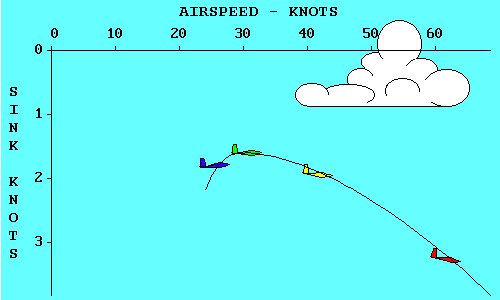Now if we connect the gliders with a smooth curve and change the distance scales to speed scales, we have just drawn a polar curve for the Schweizer SGS 1-26.

So, what can we do with a polar curve?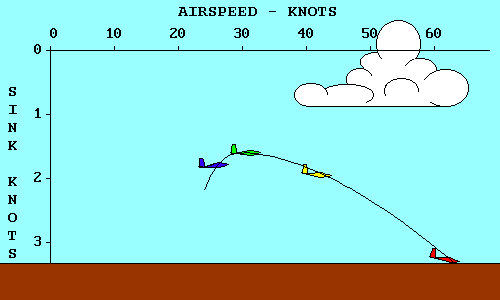Lets pretend we started this exercise with the gliders 320 feet above the ground. The red glider is already touching down (actually crashing because we didn't allow any room for a flare). If we continue the descent of the other gliders until the blue and yellow gliders also reach the surface, we see several things. First, the yellow glider goes farther than any other while losing 320 feet. The blue glider touched down about the same time, but doesn't go nearly as far. The green glider is still in the air, but will crash on top of the red glider if it isn't moved quickly.

MINIMUM SINK SPEED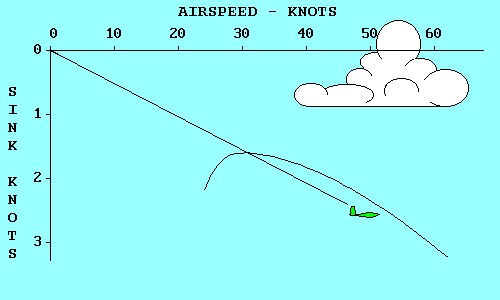Two of these gliders represent significant points on a polar curve. The green glider has the lowest sink rate of any. It will keep you in the air for the longest time, but will not go very far. Note that its glide path intersects the polar curve at its highest point. If we move up from the highest point on the polar curve to the airspeed scale we find that the minimum sink speed for a SGS 1-26 is 31 knots (35 mph). If we move horizontally to the left we find that the minimum sink rate is 1.6 knots (160 feet per minute).

BEST GLIDE SPEEDThe yellow glider has the best glide ratio of all. That means that it will glide farther in still air than any other glider, for a given loss of altitude. A line from the origin (0, 0 point) of the graph, which is tangent to (just touches) the polar curve, represents the glide path of the yellow glider. If we move up from the point where the line touches the polar curve to the airspeed scale, we find that the speed that produces this best glide is 42 knots (48 mph). If we move horizontally to the left from the tangent point we find the corresponding sink rate, 1.9 knots (190 feet per minute).
We stretched the sink scale on our graph to make it easier to read. If we had used the same scale as was used for the airspeed scale, the angle between the glider flight path and the horizontal axis would be the actual glide angle achieved by the glider. You can see that the smallest angle that can be drawn from the origin to the polar curve is the one that just touches the curve. That is why the tangent point represents the best glide speed for the glider.

If air was always still, that's all we'd need to know about polar curves. Minimum sink speed would keep us in the air for the longest time and best glide speed would allow us to go the greatest distance. Fortunately for glider pilots, air frequently moves both vertically and horizontally. When it goes up faster than our sink rate within it we can climb. When it goes down we need to modify our best glide speed to optimize our flight through sinking air.

SPEED-TO-FLY FOR DISTANCE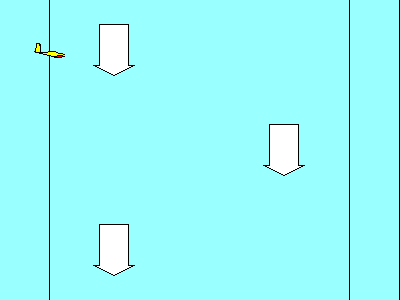To see how sinking air affects our glide let's compare the red and yellow gliders. If both enter an air mass that is sinking at 300 feet per minute (3-knots), their sink rates will increase accordingly. The yellow glider is now sinking at 4.9 kts (1.9 within the air mass plus 3 for the air mass sink rate). The red glider is now sinking at 6.2 kts (3.2 + 3.0). Both enter the air mass at the same altitude; our objective is to come out the other side with the least loss of altitude. Although the red glider is sinking faster, it is also moving horizontally much faster (62kts vs 42 kts). The result is that it gets out of the sinking air at a higher altitude than the yellow glider. The red glider has a better glide ratio, relative to the ground, than the yellow one even though the yellow glider has a better glide ratio relative to the air mass.

By now you may have noticed that the speeds for our gliders in these examples were not selected randomly. The green glider is flying at Minimum Sink Speed, the yellow glider at Best Glide Speed (also called best L/D Speed because the glide ratio in still air is numerically equal to the ratio of lift to drag), and the red glider is flying at the Speed-to-Fly for a SGS 1-26 flying in an air mass that is sinking at 300 feet per minute. For now we will define Speed-to-Fly as the speed that results in the flattest glide in both convection and wind because it was so defined by the FAA in the predecessor to the Practical Test Standards. Now let's look at how Speed-to-Fly can be determined from the polar curve for any air mass sink rate.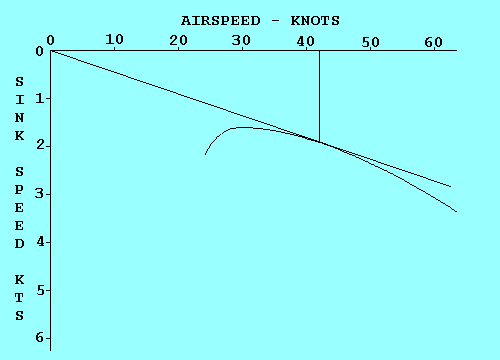In our previous examples our polar curve showed performance relative to the air mass in which the glider was flying. It was valid relative to the ground only in still air. To be valid relative to the ground the curve must be displaced in the direction and by the amount that the air mass is moving. In this example the air is sinking at 300 feet per minute (3 kts), so we move the curve down 3 kts. To determine the Speed-to-Fly for any condition, we draw a line from the origin that is just tangent to the curve. Then we draw a line straight up from the tangent point to the speed scale. In this case the Speed-to-Fly is 62 kts. Did that surprise you?

If you were trying to determine Speed-to-Fly from a polar curve drawn on a piece of paper (maybe for a pilot examiner), you would find it inconvenient to redraw the curve for each air mass sink rate.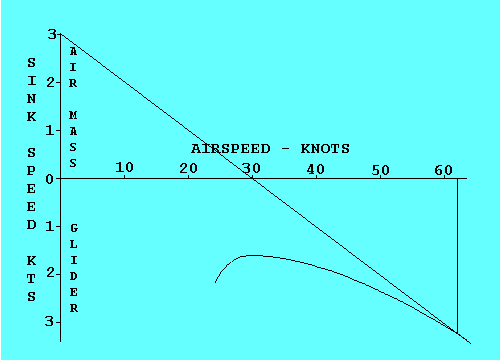An easier way to accomplish the same objective is to raise the origin of the graph by an amount equal to the air mass sink rate and then draw a tangent to the original curve from that point. To keep our examples consistent, let's assume a 3-knot air mass sink rate and move the origin up 3 knots. Now the tangent to the original curve occurs at the same 62 knots we found in the previous example. When using this method be sure to remember that the glider sink rate is the sum of its sink rate within the air mass and the sink rate of the air mass itself. In this case it is 6.2 kts (air mass sink of 3 kts plus glider sink within the air mass of 3.2 kts = 6.2 kts).

We can use the same technique to determine the Speed-to-Fly for any motion of the air mass.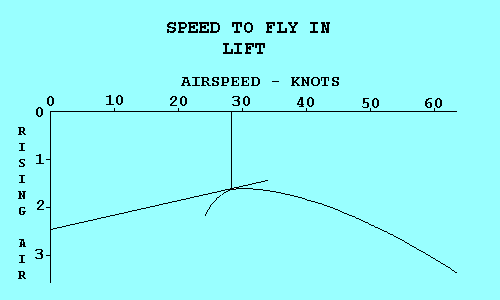If the air is rising we displace the origin down by an amount equal to the rate at which the air is going up, and then draw a tangent from that point to the polar curve. This gives a valid speed only if we plan to fly straight through the lift. If we plan to circle in the lift, other factors determine the speed that will optimize our climb rate. For a headwind we displace the origin to the right, using the airspeed scale to determine how far we move it. For a tailwind we displace the origin to the left.

We can also combine vertical and horizontal air mass movement when both exist. The example shows how to combine sink and a headwind.

You may be thinking, "This is all very interesting, but how do I use it in the air. I'm not going to carry polar curve charts with me in the glider and draw tangents for each condition I encounter." You are right. The information derived here must be converted to cockpit displays and/or rules of thumb to use in flight.

First let's discuss headwinds and tailwinds. You have to estimate their values so a rule of thumb is as good as anything. If you were to evaluate several different wind conditions graphically, you would find that the tangent point moves about half as far as the origin. Thus, a good rule would be "Add about half the estimated value of a headwind, and subtract about half the value of a tailwind from the Speed-to-Fly determined for lift or sink."

We should note here that the FAA knowledge tests ignore vertical air movement between thermals when the question relates to Speed-to-Fly. If you encounter a question about the speed to use with wind, the correct answer will be to add half the headwind to best glide speed.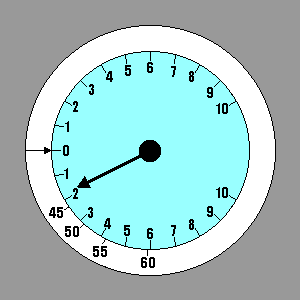But you may want to fly efficiently between thermals when the air is moving vertically, even if the FAA doesn't care. Since thermals are part of the air mass in which you are flying, they are affected by wind the same way the glider is so wind can be ignored unless you are trying to reach a point on the ground (which is not affected by the wind). An example of when you would want to take wind into consideration is trying to make it back to the airport against a headwind. Instinct probably tells you to pull back on the stick to "stretch" the glide, but the correct thing to do is push forward until the airspeed equals the speed-to-fly plus half the headwind. We have an instrument in the glider to give us the information necessary to determine speed-to-fly for lift and sink. The graphic process used to determine Speed-to-Fly gave us the sink rate of the air mass and the sink rate of the glider within the air mass. Their total is the value that would be indicated on the variometer. We could select a few representative values of Speed-to-Fly and stick them on the panel adjacent to the corresponding variometer readings, using a label maker. A better method is to invest in a speed ring for the glider/variometer combination we have. The speed ring is adjustable, but for our current definition of Speed-to-Fly it should be set with the arrow on the ring pointing to "0" on the variometer. Then when the variometer points to any rate of descent it is also pointing to the corresponding Speed-to-Fly - the one that will produce the flattest glide under those conditions. Our example shows Speed-to-Fly varying from 42 kts in still air to 62 kts in 3 kt sink.

EFFECT OF WEIGHT

The performance data used here is based on a SGS 1-26 at 575 pounds gross weight, as shown in Appendix A, The Joy of Soaring (12).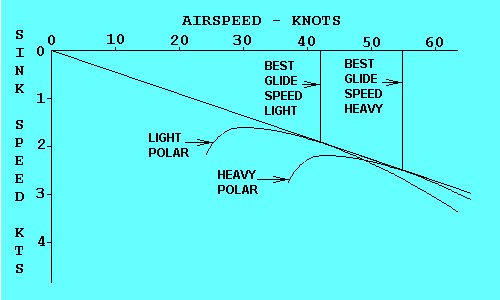A different polar curve exists for the same glider at every gross weight. As the weight increases the polar moves down and to the right and becomes a little flatter, but retains approximately the same shape. Both airspeed and sink rate increase for equivalent points on the curves, but their ratio remains the same. Both heavy and light gliders achieve the same best glide ratio, as shown here, but the heavy glider does so at a higher speed. This is the reason for carrying ballast to improve glide performance when the thermals are strong enough, even though the climb rate suffers.

The formula for the lift generated by a wing is: L = CL x d/2 x S x V2, where L is the lift force, CL is the coefficient of lift (directly proportional to the angle of attack if the wing is not stalled), "d" is the density of the air, S is the wing area, and V is the airspeed. The formula for total drag is D = CD x d/2 x S x V2, where D is the total drag force, CD is the coefficient of total drag equal to the sum of the coefficients of parasite and induced drag, and the other terms are the same as in the lift formula. The formula for the coefficient of total drag is CD = C0 + CL2/ (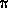x A x e). C0 is the coefficient of parasite drag and is essentially constant. The remaining expression shows that induced drag is highly dependent on the coefficient of lift (high angle of attack usually at low airspeed) and aspect ratio (A). "e" is there to account for other factors, but its value is usually near 1 for gliders. Don't panic; you are not required to memorize these formulae.

The message you need to get is that both lift and drag depend on air density and airspeed and two factors associated with the specific glider, wing area, and coefficient of lift. When weight increases, lift must increase proportionately. If we are to fly the glider in the same air mass (e.g. same density), with the same wing area, and at the most efficient coefficient of lift, we must increase the airspeed. When we do, the drag increases in the same proportion as lift, so the lift/drag ratio remains the same.

EFFECT OF TURNING

The increase in load factor that accompanies turning flight is equivalent to an increase in weight with respect to performance airspeeds. The polar curve is effectively displaced down and to the right. The most significant aspect of this shift is that the minimum sink speed is higher when turning (e.g. when thermalling) than it is in straight flight. This increase, as with stall speed, is proportional to the square root of load factor, and equals a 4l% increase at a 60-degree bank.

We should note that the glider performance graphs used with the FAA knowledge tests (shown below) are not presented in the same format as those in our illustrations. The curve labeled "GLIDE ANGLE" looks like a polar curve, but it is not. It is derived from a polar curve and allows direct measurement of glide ratio (not really glide angle). The curve labeled "SINKING SPEED" is essentially the same as the polar curves we have been using except that it is inverted. Some engineers prefer it that way but many glider pilots find the version that shows increasing sink down rather than up to be a more logical presentation. If you wanted to find the best glide speed graphically from this graph you would have to extend both scales back to zero, since that is where the tangent line must start.

The new FAA publication, "Glider Flying Handbook"(18), illustrates polar curves in the same way that we have done so here, so some future version of the knowledge test may do so as well. In the meantime, check the illustrations carefully for how they are labeled. Similar curves do not always represent the same information.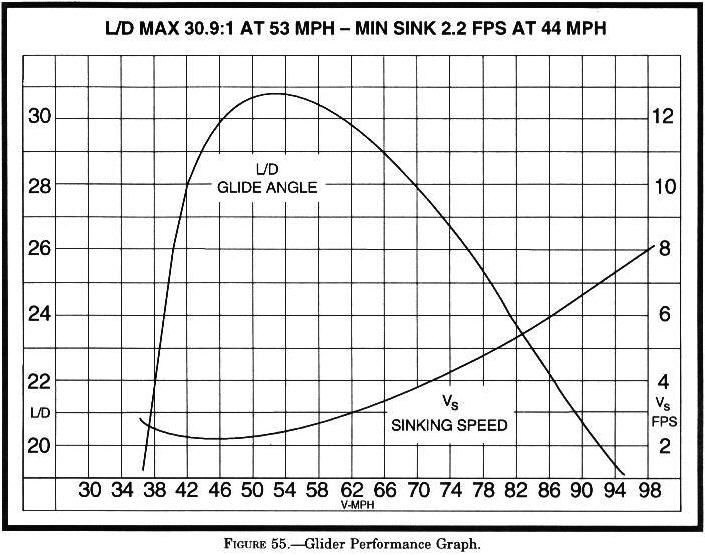© 2005 Jim D. Burch 602-942-2734 jdburch@att.net